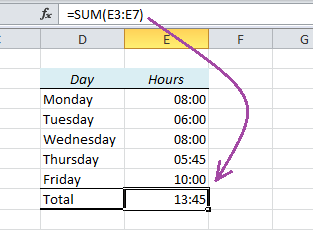# How to Summarize Hours in Excel and Show the Right ResultSometimes Excel doesn’t seem to add up the hours correctly. In this table I have made a table of an employee’s working hours and used the SUM function to add them together. Look what happens:

The sum is 13:45 instead of the expected 37:45. That’s 24 hours less than we wanted!

Here’s why: The result is actually 1 day, 13 hours and 45 minutes, but when you use the SUM function, Excel assumes that you want to keep the same format as above, which only shows hours and minutes.

How to fix it: